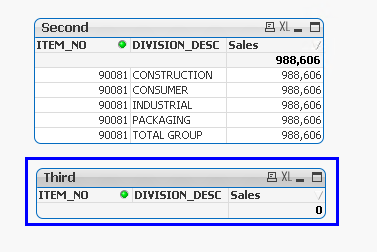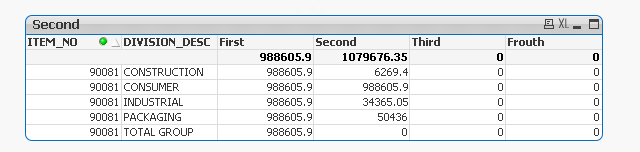Announcements
You can succeed best and quickest by helping others to succeed. Join the conversation.
cancel
Showing results for
Did you mean:Creator II

## Nested Aggr() expression in Table.

Hello All,

i have scenario as below.

i have two table as shown in below image.For "First" table i used below expression.

Aggr(sum({< DIVISION_DESC-={'TOTAL GROUP'},Accounts={'Gross sales'},REC_TYPE={InvoiceDateRec}>}RAGLDA_ACCTD_AMOUNT*XRATE_CONST*RATE), ITEM_NO,DIVISION_DESC)

and for "Second" table i used below expression.

(Aggr(Max((Aggr(sum({< DIVISION_DESC-={'TOTAL GROUP'},Accounts={'Gross sales'},REC_TYPE={InvoiceDateRec}>}

RAGLDA_ACCTD_AMOUNT*XRATE_CONST*RATE), ITEM_NO,DIVISION_DESC))),ITEM_NO))

what i want to do here is, i want to take Max Sales line but if you observe "First" and "Second" table closely in second table that "Division_Desc" column is wrong because in "First" table we have "CONSUMER" but in second table somehow it is taking "INDUSTRIAL".

Desire Result:-

i need final result as

ITEM_NO     DIVISION_DESC         SALES

90081            CONSUMER             988,606

Thanks in Advance.1 Solution

Accepted SolutionsMVP

May be a rounding problem... try this for the third expression and see what you get

If(

Floor(Max(TOTAL <ITEM_NO> Aggr(Sum({< DIVISION_DESC-={'TOTAL GROUP'},Accounts={'Gross sales'},REC_TYPE={InvoiceDateRec}>}RAGLDA_ACCTD_AMOUNT*XRATE_CONST*RATE), ITEM_NO,DIVISION_DESC)))

=

Floor(sum({< DIVISION_DESC-={'TOTAL GROUP'},Accounts={'Gross sales'},REC_TYPE={InvoiceDateRec}>}RAGLDA_ACCTD_AMOUNT*XRATE_CONST*RATE))

, 1, 0)

8 RepliesMVP

May be try this

If(Max(TOTAL <ITEM_NO> Aggr(Sum({< DIVISION_DESC-={'TOTAL GROUP'},Accounts={'Gross sales'},REC_TYPE={InvoiceDateRec}>}RAGLDA_ACCTD_AMOUNT*XRATE_CONST*RATE), ITEM_NO,DIVISION_DESC) = sum({< DIVISION_DESC-={'TOTAL GROUP'},Accounts={'Gross sales'},REC_TYPE={InvoiceDateRec}>}RAGLDA_ACCTD_AMOUNT*XRATE_CONST*RATE), sum({< DIVISION_DESC-={'TOTAL GROUP'},Accounts={'Gross sales'},REC_TYPE={InvoiceDateRec}>}RAGLDA_ACCTD_AMOUNT*XRATE_CONST*RATE))Creator II
Author

i have paste below expression as per your suggestion, but it is not returning any row.

If(Max(TOTAL <ITEM_NO> Aggr(Sum({< DIVISION_DESC-={'TOTAL GROUP'},Accounts={'Gross sales'},REC_TYPE={InvoiceDateRec}>}RAGLDA_ACCTD_AMOUNT*XRATE_CONST*RATE), ITEM_NO,DIVISION_DESC))

= sum({< DIVISION_DESC-={'TOTAL GROUP'},Accounts={'Gross sales'},REC_TYPE={InvoiceDateRec}>}RAGLDA_ACCTD_AMOUNT*XRATE_CONST*RATE),

sum({< DIVISION_DESC-={'TOTAL GROUP'},Accounts={'Gross sales'},REC_TYPE={InvoiceDateRec}>}RAGLDA_ACCTD_AMOUNT*XRATE_CONST*RATE))MVP

Lets break it down

1) Max(TOTAL <ITEM_NO> Aggr(Sum({< DIVISION_DESC-={'TOTAL GROUP'},Accounts={'Gross sales'},REC_TYPE={InvoiceDateRec}>}RAGLDA_ACCTD_AMOUNT*XRATE_CONST*RATE), ITEM_NO,DIVISION_DESC))

Should give you 988,606 for all the rows

2) sum({< DIVISION_DESC-={'TOTAL GROUP'},Accounts={'Gross sales'},REC_TYPE={InvoiceDateRec}>}RAGLDA_ACCTD_AMOUNT*XRATE_CONST*RATE)

Should give individual sums for each row

3) If(Max(TOTAL <ITEM_NO> Aggr(Sum({< DIVISION_DESC-={'TOTAL GROUP'},Accounts={'Gross sales'},REC_TYPE={InvoiceDateRec}>}RAGLDA_ACCTD_AMOUNT*XRATE_CONST*RATE), ITEM_NO,DIVISION_DESC)) = sum({< DIVISION_DESC-={'TOTAL GROUP'},Accounts={'Gross sales'},REC_TYPE={InvoiceDateRec}>}RAGLDA_ACCTD_AMOUNT*XRATE_CONST*RATE), 1, 0)

Should give you 1 for the max row and 0 for all others

4) If(Max(TOTAL <ITEM_NO> Aggr(Sum({< DIVISION_DESC-={'TOTAL GROUP'},Accounts={'Gross sales'},REC_TYPE={InvoiceDateRec}>}RAGLDA_ACCTD_AMOUNT*XRATE_CONST*RATE), ITEM_NO,DIVISION_DESC)) = sum({< DIVISION_DESC-={'TOTAL GROUP'},Accounts={'Gross sales'},REC_TYPE={InvoiceDateRec}>}RAGLDA_ACCTD_AMOUNT*XRATE_CONST*RATE), sum({< DIVISION_DESC-={'TOTAL GROUP'},Accounts={'Gross sales'},REC_TYPE={InvoiceDateRec}>}RAGLDA_ACCTD_AMOUNT*XRATE_CONST*RATE), 0)

Should give you the max on the max row and give 0 otherwise...

Can you post the screenshot of what you get using the above 4 expressionsCreator II
Author

Hello Sunny,

First two are behaving as you mentioned but Third Expression is not returning any row at all.MVP

I asked all four expression in a single chart? What is the above screenshot? Don't understand where was the miss communication my friend?Creator II
Author

Sorry for that,

Please find below screenshot with all four exp. in one chart.MVP

May be a rounding problem... try this for the third expression and see what you get

If(

Floor(Max(TOTAL <ITEM_NO> Aggr(Sum({< DIVISION_DESC-={'TOTAL GROUP'},Accounts={'Gross sales'},REC_TYPE={InvoiceDateRec}>}RAGLDA_ACCTD_AMOUNT*XRATE_CONST*RATE), ITEM_NO,DIVISION_DESC)))

=

Floor(sum({< DIVISION_DESC-={'TOTAL GROUP'},Accounts={'Gross sales'},REC_TYPE={InvoiceDateRec}>}RAGLDA_ACCTD_AMOUNT*XRATE_CONST*RATE))

, 1, 0)Creator II
Author

Yes, now its is working as per expected.

Thanks a lot Sunny. you are champ.Community Browser